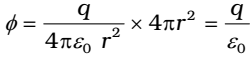# Gauss Law – Gauss Theorem Formula, Significance

0
173

## Gauss Law

The surface integral of electrostatic field produced by any source over any closed surface S enclosing a volume V in vacuum i.e. total electric flux over the closed surface S in vacuum is 1/epsilon times the total charge Q contained inside S.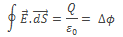The surface chosen to calculate the surface integral is called Gaussian surface.

## Gaussian Theorem

The flux through area element ∆S is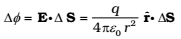The unit vector rˆ is along the radius vector from the centre to the area element. Now, since the normal to a sphere at every point is along the radius vector at that point, the area element ΔS and rˆ have the same direction. Therefore,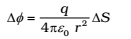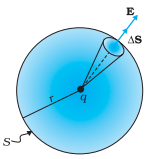Since the magnitude of a unit vector is 1. The total flux through the sphere is obtained by adding up flux through all the different area elements:Since each area element of the sphere is at the same distance r from the charge,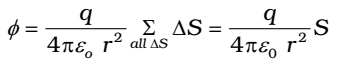Now S, the total area of the sphere, equals 4πr2 . Thus,